# How to Use the COLUMNS Function in Excel

The COLUMNS function returns the number of COLUMNS in an array or cell reference in Excel. Unlike the COLUMN function which returns the particular column number of the cell reference.
Syntax:

=COLUMNS(array)

Let’s run some tests on using the COLUMNS function Excel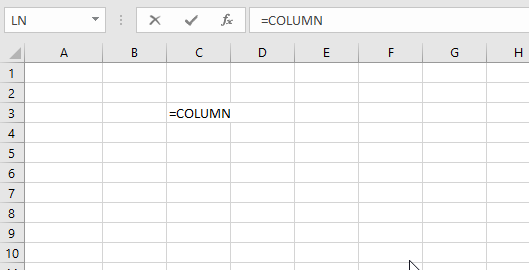As you can see, we selected 3 rows & 2 Columns in the array. So it returns 2 as number of columns in the array.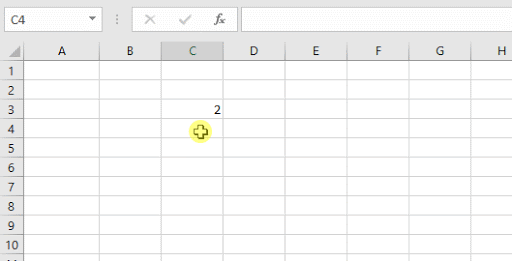As you can see here the array has only 1 column. So it returns 1 the count of COLUMNS in the array.
Here we selected a little larger array to get the count the columns in the table.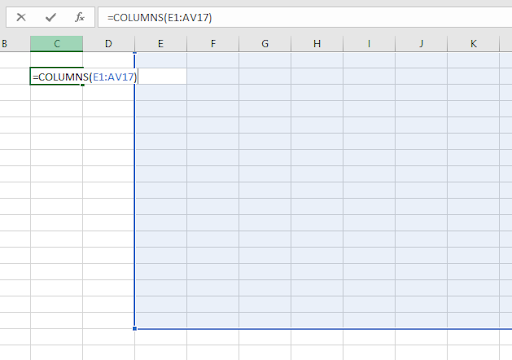Press Enter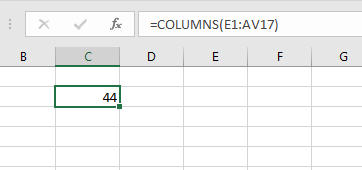As you can see the count of COLUMNS in the above array is 44.
You can use the COLUMNS function in Excel without using cell references.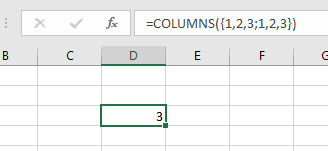Excel will read this like array has 2 rows and 3 columns. The values along the columns are separated using commas (,) and values of different rows separated using semicolon(;) like shown below.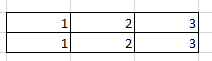You can use the COLUMNS function with other excel functions.

As you can see from the above examples that how to use COLUMNS function in Excel.

Hope you understood how to use the COLUMNS function in Excel. Explore more articles on Excel cell reference function here. Please state your queries in the comment box below.

Related Articles:

How to Use the COLUMN Function in Excel

How to use the Excel ROW function

How to use the ROWS function in Excel

Popular Articles:

50 Excel Shortcuts to Increase Your Productivity

How to use the VLOOKUP Function in Excel

How to use the COUNTIF in Excel 2016

How to use the SUMIF Function in Excel

Terms and Conditions of use

The applications/code on this site are distributed as is and without warranties or liability. In no event shall the owner of the copyrights, or the authors of the applications/code be liable for any loss of profit, any problems or any damage resulting from the use or evaluation of the applications/code.